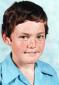# Full and nested submodel comparisons

6 posts / 0 newOffline
Joined: 09/24/2009 - 15:44
Full and nested submodel comparisons

Hello All,
Based on the generic script (below) from the OpenMx webpage I'm testing a very simple sub-model in which I drop one element of the 'S' matrix to zero and then compare back to the full model. I get an error message which says "Error in parameters[[i]] : subscript out of bounds". Does anyone have an idea as to why this might be happening?
Cheers,
Nathan

require(OpenMx)
data(demoOneFactor)
manifests <- names(demoOneFactor)
latents <- c("G")
factorModel <- mxModel("One Factor", type="RAM",
manifestVars = manifests,
latentVars = latents,
mxPath(from=latents, to=manifests),
mxPath(from=manifests, arrows=2),
mxPath(from=latents, arrows=2,
free=F, values=1.0),
mxData(cov(demoOneFactor), type="cov",
numObs=500))
summary(mxRun(factorModel))

factorModelFit <- mxRun(factorModel) # Run model
factorModelFitSumm <- summary(factorModelFit)

# Drop element [5,5] to 0 in S matrix

factorModelFit@matrices$S@free[5,5] <- F factorModelFit@matrices$S@values [5,5] <- 0

sub1 <-mxRun(factorModelFit)

# Compare Full and Nested models

tableFitStatistics(factorModelFit,sub1)Offline
Joined: 07/31/2009 - 15:24
I know why the error is

I know why the error is occurring. The summary() function is using the free parameter estimates from optimization. By deleting a free parameter after a model is run, the length of the free parameter list is no longer accurate.

This is relatively easy to fix. But also the same bug with standard error estimates needs to be fixed. Fixing the bug in the library will not happen tonight.

As a workaround, use the following for now:

factorModel$S@free[5,5] &lt;- F factorModel$S@values[5,5] &lt;- 0
sub1 &lt;- mxRun(factorModel)
tableFitStatistics(factorModelFit, sub1)Offline
Joined: 07/31/2009 - 15:24
OK. So in a branch of the

OK. So in a branch of the source code repository I've committed an update to the summary() function. The new function stores the model state immediately after optimization, and uses that information to produce summary output.

This change will be merged into the OpenMx trunk after the Twins Workshop. Still TODO: fix the summary statistics for independent submodels.Offline
Joined: 09/24/2009 - 15:44
Thanks for that.

Thanks for that. Unfortunately, I still get the same message: "Error in parameters[[i]] : subscript out of bounds" even when I write out a sub model in full. I'll wait for the update. Cheers, Nathan

require(OpenMx)
data(demoOneFactor)
manifests <- names(demoOneFactor)
latents <- c("G")
factorModel <- mxModel("One Factor", type="RAM",
manifestVars = manifests,
latentVars = latents,
mxPath(from=latents, to=manifests),
mxPath(from=manifests, arrows=2),
mxPath(from=latents, arrows=2,
free=F, values=1.0),
mxData(cov(demoOneFactor), type="cov",
numObs=500))
summary(mxRun(factorModel))

factorModelFit <- mxRun(factorModel) # Run model
factorModelFitSumm <- summary(factorModelFit)

# ---

require(OpenMx)
data(demoOneFactor)
manifests <- names(demoOneFactor)
latents <- c("G")
sub1 <- mxModel("One Factor", type="RAM",
manifestVars = manifests,
latentVars = latents,
mxPath(from=latents, to=manifests),
mxPath(from=manifests, arrows=2),
mxPath(from=latents, arrows=2,
free=F, values=1.0),
mxData(cov(demoOneFactor), type="cov",
numObs=500))

# Drop element [5,5] to 0 in S matrix

factorModel$S@free[5,5] <- F factorModel$S@values [5,5] <- 0

summary(mxRun(sub1))

sub1Fit <- mxRun(sub1) # Run model
sub1FitSumm <- summary(sub1Fit)

# Compare Full and Nested models

tableFitStatistics(factorModelFit,sub1Fit)Offline
Joined: 07/31/2009 - 15:24
Umm, I ran the second program

Umm, I ran the second program and it worked. I re-installed the 0.2.6-1114 binary to make sure I'm not running a patched copy. The first program will work if you eliminate the free parameter in factorModel instead of factorModelFit.

Also you don't want to use:

factorModel$S@free[5,5] <- F factorModel$S@values[5,5] <- 0

if you're going to redefine the sub1 model. In that case you would need:

sub1$S@free[5,5] <- F sub1$S@values[5,5] <- 0Offline
Joined: 07/31/2009 - 14:25
Just so people have a script

Just so people have a script that works here...

require(OpenMx)
source("http://www.vipbg.vcu.edu/~vipbg/Tc24/GenEpiHelperFunctions.R")
data(demoOneFactor)
manifests = names(demoOneFactor)
latents = c("G")
factorModel = mxModel("One Factor",
type="RAM",
manifestVars = manifests,
latentVars = latents,
mxPath(from = latents, to=manifests),
mxPath(from = manifests, arrows=2),
mxPath(from = latents, arrows=2,free = F, values=1.0),
mxData(cov(demoOneFactor), type = "cov",numObs = 500)
)
fit = mxRun(factorModel)

# --- try a submodel dropping one path to zero and comparing fit

sub1 = mxModel(factorModel, name="reduced")

# Drop element [1,6] in the A(symmetric path) matrix

sub1$A@free[1,6] = F sub1$A@values [1,6] = 0
fit2 = mxRun(sub1)

# Compare Full and Nested models

tableFitStatistics(fit,fit2) # function in the GenEpiHelperFunctions.R library
fit2\$A # examine the dropped matrix
omxGraphviz(model=fit2, dotFilename='test.dot') # view diagram if you have a .dot viewer
system('open test.dot');